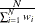The SEVERITY Procedure

WEIGHT Statement

• WEIGHT variable-name;

The WEIGHT statement specifies the name of a variable whose values represent the weight of each observation. PROC SEVERITY associates a weight of w to each observation, where w is the value of the WEIGHT variable for the observation. If the weight value is missing or less than or equal to 0, then the observation is ignored and a warning is written to the SAS log. When you do not specify the WEIGHT statement, each observation is assigned a weight of 1. If you specify more than one WEIGHT statement, then the last statement is used.

The weights are normalized so that they add up to the actual sample size. In particular, the weight of each observation is multiplied by, where N is the sample size.